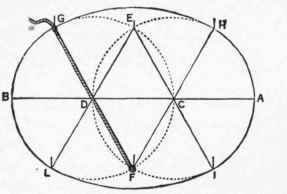## Circles

The diameter of any circle being known, the circumference is obtained by multiplying it by 31416 or 22/7. Thus, a circle with a diameter of 12 in. would have a circumference of 37.6992 in. The radius of a circle is half the diameter. The area of a circle is obtained either (1) by squaring the diameter and multiplying by .7854 or (2) by multiplying the square of the radius by 3.1416. The following are useful formulae relating to the circle: -

Circumference of circle x . .3183 = diameter.

„ „ X 915 = radius.

„ „ x 1.2732 = square of diameter.

„ „ x 12.5663 = square of the circumference.

„ „ x .2756 = side of an inscribed equilateral triangle.

„ „ / 3.1416 = diameter.

„ „ / 6.2831 = radius.

## Oval, Ellipse

These are two common shapes for flower beds in gardens. The methods used for striking ovals and ellipses in gardens are shown in the accompanying diagrams (figs. 406, 407). In the first one it will be seen that two intersecting circles are made, one with the centre at D, the other at C - both equal. The gap between the two is filled in by freehand. To find the area of an ellipse multiply the long axis by the short axis, and the product by .7854. The same result may be secured by multiplying half the long or main axis by half the short axis, and the product by 31416.

Having decided on the length of an elliptical bed, the most simple method of striking the figure is to fix two pegs at equal distances in from the ends of the long axis. Then make a string loop equal to the entire length of the long axis and place to enclose the two pegs. By stretching the loop tightly with a pointed stick, and working right round from one end of the long axis to the starting point, the form of an ellipse may be traced on the ground.

## Cone

The area equals half the product of the circumference of base multiplied by the slant height. Example: The radius of the base of a cone is 8 in. and the slant height 14 in. Circumference of base = 2x8 X 31416 = 50.2656; and area = 1/2 x 14 x 502656 = 3518592 sq. in.Fig. 406. - Showing how an Oval Bed is struck from 2 circles, and how the intervening space may be filled in.Fig. 407. - Showing how to make an Elliptical Bed.

The rule for finding the area of the surface of a cone may be used for estimating the approximate extent of the leaf surface of many kinds of trees, shrubs, and bushes, which are often conical in shape. Thus a tree or bush having a spread of 10 ft. across the base of the branches, and a slant height of 10 ft. from base to apex, would have an approximate leaf surface of (10x3.1416x10)/2= 157.08 sq. ft.

Most flower pots are cones with the ends cut off - in other words, a flower pot is a frustum of a cone. To find the area of the curved surface of a flower pot, multiply the sum of the circumferences of the two ends of the flower pot (frustum) by the slant height of the pot (frustum), and half the product will be the area of the curved surface.

Example: The radius of the bottom or narrow end of a flower pot is 10 in., and the radius of the top or broad end is 15 in.; the slant height is 16 in. Find the area of the curved surface.

The sum of the circumferences in inches is the product of 3.1416 into the sum of 20 and 30, i.e. into 50. Thus the sum of the circumferences is 50 x 3.1416 in. Multiply this sum by the slant height, 16, and divide by 2, thus: (16x50x3.1416) /2= 8x50x 3.1416 = 400 X 31416 = 125664 sq. in.